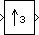# Upsample

Resample input at higher rate by inserting zeros

• Library:
• DSP System Toolbox / Signal Operations

DSP System Toolbox HDL Support / Signal Operations

•## Description

The Upsample block resamples each channel of the Mi-by-N input at a rate L times higher than the input sample rate by inserting L-1 zeros between consecutive samples. Specify the integer L in the Upsample factor parameter. Use the Sample offset parameter D to delay the output samples by a specific number of sample periods. Doing so enables you to select any of the L possible output phases. The value you specify in the Sample offset parameter must be an integer in the range $0\le D<\left(L-1\right)$.

You can use this block inside triggered subsystems when you set the Rate options parameter to ```Enforce single-rate processing```.

## Ports

### Input

expand all

Specify the input signal as a real or a complex-valued scalar, vector, or a matrix signal.

When you set Input processing to `Columns as channels (frame based)` and Rate options to ```Enforce single-rate processing```, the input can be a variable-size signal. That is, the frame size (number of rows) and the number of channels (columns) of the signal can change during simulation.

Data Types: `single` | `double` | `int8` | `int16` | `int32` | `uint8` | `uint16` | `uint32` | `Boolean` | `fixed point`
Complex Number Support: Yes

### Output

expand all

The Upsample block outputs a signal that has the same data type and complexity as the input signal. The size of the output depends on the value of the Upsample factor, L parameter and the setting of the Rate options parameter.

If Upsample factor is set to L and Rate options is set to:

• `Enforce single-rate processing` –– The block maintains the input sample rate by increasing the output frame size by a factor of L.

• `Allow multirate processing` –– The block resamples the signal such that the output sample rate is L times faster than the input sample rate. The output signal has the same frame size as the input signal.

When the input is a variable-size signal, the output is also a variable-size signal.

Data Types: `single` | `double` | `int8` | `int16` | `int32` | `uint8` | `uint16` | `uint32` | `Boolean` | `fixed point`
Complex Number Support: Yes

## Parameters

expand all

Specify the upsample factor L by which to increase the input sample rate as a positive integer.

Specify the sample offset D as an integer in the range [0, L−1].

Specify how the block should process the input. You can set this parameter to one of these options:

• `Columns as channels (frame based)` — When you select this option, the block treats each column of the input as a separate channel. In this mode, the block can perform single-rate or multirate processing.

• `Elements as channels (sample based)` — When you select this option, the block treats each element of the input as a separate channel. In this mode, the block always performs multirate processing.

Specify the method by which the block upsamples the input. You can select one of the following options:

• `Enforce single-rate processing` — When you select this option, the block maintains the input sample rate by increasing the output frame size by a factor of L. To select this option, you must set the Input processing parameter to ```Columns as channels (frame based)```.

• `Allow multirate processing` — When you select this option, the block resamples the signal such that the output sample rate is L times faster than the input sample rate.

Specify the value with which the block is initialized for cases of nonzero latency as a scalar, vector, or a matrix. The scalar value appears in the output as the sample D+1. If this parameter is set to a vector or a matrix, the size of this parameter should be the same as that of the input. This parameter appears only when you configure the block to perform multirate processing.

#### Dependencies

To enable this parameter, set Rate options to `Allow multirate processing`.

## Block Characteristics

 Data Types `Boolean` | `double` | `fixed point` | `integer` | `single` Direct Feedthrough `no` Multidimensional Signals `no` Variable-Size Signals `no` Zero-Crossing Detection `no`

expand all

## Version History

Introduced before R2006a

expand all